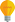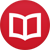# Differentiation (derivatives) without variables

by Jakub MarianTip: See my list of theMost Common Mistakes in English. It will teach you how to avoid mis­takes with com­mas, pre­pos­i­tions, ir­reg­u­lar verbs, and much more.

To be able to differentiate a function without using variables, we have to be able to denote the function we want to differentiate without variables first. I have written a separate article on the topic which you might want to read first. Just to remind you: $\iota$ denotes the identity function $\iota: ℝ → ℝ$, $\iota(x) = x$. Using this function, we are able to denote any function $f: ℝ → ℝ$ that we are able to denote using variables; just replace the variable by $\iota$. However, there are many new constructions we can now use; for example we can write

$$({\log}+\iota^2+÷{1}{\iota})∘({\cos}+{\sin}+\iota)$$

for what would be traditionally written as

$$\log(\cos(x)+\sin(x)+x)+(\cos(x)+\sin(x)+x)^2+÷{1}{\cos(x)+\sin(x)+x}\,,$$

and you can imagine how the latter expression would expand if more than two functions were composed. Our goal now will be to learn how to translate the process of differentiation into this language. We will denote the derivative by the “prime” symbol, i.e. the derivative of $f$ is $f’$.

Arithmetical rules look pretty much the same, i.e. $(f+g)’ = f’+g’$ and $(fg)’ = f’g+fg’$. Only the chain rule has two different variants because we use the notations $f[g]$ and $f ∘ g$ interchangeably:

$$(f∘g)’ = (f[g])’ = f’[g]g’ = (f’∘g)g’\,.$$

It should be clear, hopefully, how to translate formulas for derivatives of elementary functions into the notation without variables, for example:

\begin{align*} \arctan’ &= ÷{1}{1+\iota^2} \\ \log’ &= ÷{1}{\iota} \\ \tan’ &= ÷{1}{\cos^2}\,. \end{align*}

From the pedagogical point of view, this notation makes applying the rules of differentiation in practice much easier for students, for example, $$\l(\arctan[{\sin}+{\cos}]\r)’ = \l(÷{1}{1+\iota^2}[{\sin}+{\cos}]\r)({\cos}-{\sin}) = ÷{{\cos}-{\sin}}{1+({\sin}+{\cos})^2}\,,$$

or

\begin{align*} ({\sin^3}∘\cos^4)’ &= (\iota^3∘{\sin}∘\iota^4∘\cos)’ \\ &= (3\iota^2∘{\sin}∘\iota^4∘\cos)({\cos}∘\iota^4∘\cos)(4\iota^3∘\cos)(-\sin) \\ &= (3{\sin^2}∘\cos^4)({\cos}∘\cos^4)(4\cos^3)(-\sin)\,, \end{align*}

(i.e. the function traditionally denoted $\sin^3(\cos^4(x))$. The approach also has undeniable practical value, not just pedagogical; let’s differentiate a more complicated function that would be traditionally written as

$$f(x) = \frac{(\sin x+\cos x)^3+e^{\sin x + \cos x}}{1+(\sin x + \cos x)^4}\,.$$

In our notation, we can notice that the function actually has a simpler structure:

$$f = \frac{({\sin}+{\cos})^3+e^{{\sin}+{\cos}}}{1+({\sin}+{\cos})^4} = \frac{\iota^3+e^\iota}{1+\iota^4}[{\sin}+{\cos}]\ ,$$

and so we would differentiate it in this form:

$$f’ = \l(\frac{(3\iota^2+e^\iota)(1+\iota^4)-(\iota^3+e^\iota)4\iota^3}{(1+\iota^4)^2}[\sin+\cos]\r)(\cos-\sin)\,.$$

This could, of course, be further simplified, but it is nevertheless a complete result. There is no way to “notice” the simpler structure in the traditional notation because there is no convenient way to denote it; thus we are forced to repeat the same step many times:

\begin{align*} &\left(\frac{(\sin(x)+\cos(x))^3+e^{\sin(x) + \cos(x)}}{1+(\sin(x) + \cos(x))^4}\right)’ = \Big(\big(3(\sin(x)+\cos(x))^2(\cos(x)-\sin(x))+ \\ &{}+e^{\sin(x) + \cos(x)}\cdot(\cos(x) -\sin(x))\big)(1+(\sin(x)+\cos(x))^4)-\big((\sin(x)+\cos(x))^3 \\ &{}+e^{\sin(x) + \cos(x)}\big)\cdot4(\sin(x)+\cos(x))^3(\cos(x)-\sin(x))\Big)/\big(1+(\sin(x)+\cos(x))^4\big)^2\,. \end{align*}

Further algebraical manipulations with such an expression would be very complicated, and its structure is unclear. Also, in terms of computational complexity, the first expression is very friendly because the value at a particular point (e.g. 0) can be computed quite simply:

\begin{align*} f’(0) &= \frac{(3\iota^2+e^\iota)(1+\iota^4)-(\iota^3+e^\iota)4\iota^3}{(1+\iota^4)^2}\l(\sin(0)+\cos(0)\r)\,(\cos(0)-\sin(0)) \\ &= \frac{(3\iota^2+e^\iota)(1+\iota^4)-(\iota^3+e^\iota)4\iota^3}{(1+\iota^4)^2}(1) = \frac{(3+e)(1+1)-(1+e)4}{2^2} \\ &= \frac{1-e}{2}\,. \end{align*}

An algorithm computing the value using the traditionally written expression would require much more time (because it would be necessary to repeat the most time-consuming steps many times).

By the way, I have written several educational ebooks. If you get a copy, you can learn new things and support this website at the same time—why don’t you check them out?